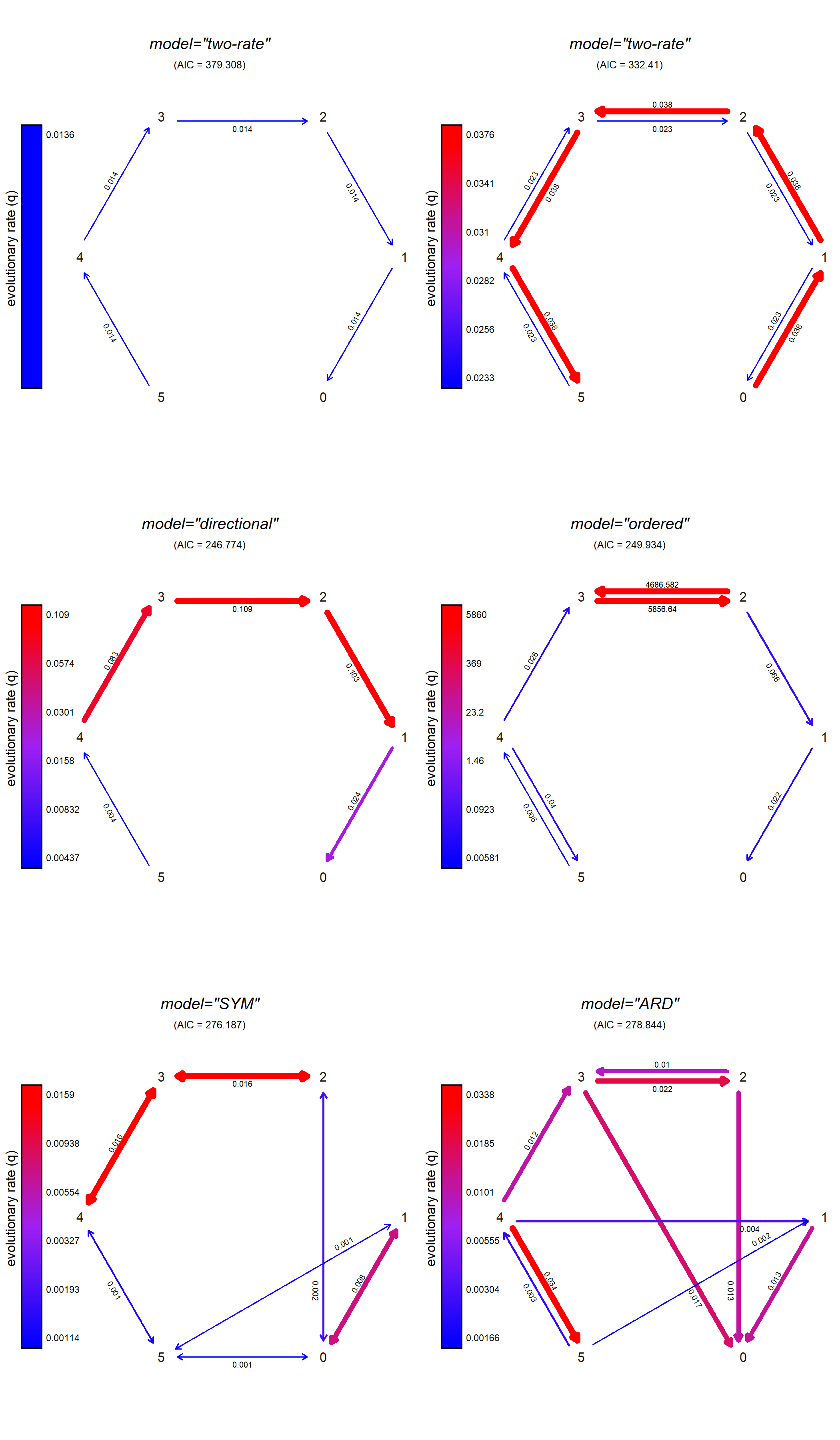## Tuesday, October 25, 2022

### Fix to d.f. from `fitMk.parallel` and `fitMk(...,opt.method="optimParallel")`

Last week, Luke Harmon and I taught a phylogenetic comparative methods in R workshop at the University of Wyoming – in part to celebrate the recent launch of our book of the same name.

One of our discoveries during the workshop was that the new phytools parallelized Mk model-fitting function, `fitMk.parallel` (which can now be called as an option of `fitMk`) had a bug were it was not returning the correct degrees of freedom for its corresponding `logLik` method. This made the `AIC` and `anova` methods also malfunction, since they depend on `logLik`.

This has now been fixed.

Here's a quick demo, using the same example (squamate digit number evolution with data from Brandley et al. 2008) as in our book.

``````library(phytools)
packageVersion("phytools")
``````
``````##  '1.2.8'
``````
``````library(geiger)
## read tree and data from file
row.names=1)
## pull out trait vector
toes<-as.factor(setNames(sqData[],rownames(sqData)))
## subsample tree and data to match
chk<-name.check(sqTree,sqData)
summary(chk)
``````
``````## 139 taxa are present in the tree but not the data:
##     Abronia_graminea,
##     Acontias_litoralis,
##     Acontophiops_lineatus,
##     Acrochordus_granulatus,
##     Agamodon_anguliceps,
##     Agkistrodon_contortrix,
##     ....
## 1 taxon is present in the data but not the tree:
##     Trachyboa_boulengeri
##
## To see complete list of mis-matched taxa, print object.
``````
``````sqTree<-drop.tip(sqTree,chk\$tree_not_data)
toes<-toes[sqTree\$tip.label]
``````

Now let's fit six models. In order, I'll fit: (1) a one-rate, digit loss only model (d.f. = 1); (2) a two-rate, gain & loss model (d.f. = 2); (3) a directional, multi-rate loss-only model (d.f. = 5); (4) an ordered, multi-rate, gain & loss model (d.f. = 10); (5) a symmetric model (d.f. = 15); and, finally (6) an all-rates-different model (d.f. = 30).

``````## fit one-rate loss-only model
onerate<-matrix(c(
0,0,0,0,0,0,
1,0,0,0,0,0,
0,1,0,0,0,0,
0,0,1,0,0,0,
0,0,0,1,0,0,
0,0,0,0,1,0),6,6,byrow=TRUE,
dimnames=list(levels(toes),levels(toes)))
fitOneRate<-fitMk(sqTree,toes,model=onerate,pi="fitzjohn",
opt.method="nlminb",logscale=TRUE)

## fit two-rate gain(1) & loss(2) model
tworate<-matrix(c(
0,1,0,0,0,0,
2,0,1,0,0,0,
0,2,0,1,0,0,
0,0,2,0,1,0,
0,0,0,2,0,1,
0,0,0,0,2,0),6,6,byrow=TRUE,
dimnames=list(levels(toes),levels(toes)))
fitTwoRate<-fitMk(sqTree,toes,model=tworate,pi="fitzjohn",
opt.method="optimParallel")

## fit loss-only multi-rate model
directional<-matrix(c(
0,0,0,0,0,0,
1,0,0,0,0,0,
0,2,0,0,0,0,
0,0,3,0,0,0,
0,0,0,4,0,0,
0,0,0,0,5,0),6,6,byrow=TRUE,
dimnames=list(levels(toes),levels(toes)))
fitDirectional<-fitMk(sqTree,toes,model=directional,
pi="fitzjohn",opt.method="nlminb",logscale=TRUE)

## fit loss/gain multi-rate model
ordered<-matrix(c(
0,1,0,0,0,0,
2,0,3,0,0,0,
0,4,0,5,0,0,
0,0,6,0,7,0,
0,0,0,8,0,9,
0,0,0,0,10,0),6,6,byrow=TRUE,
dimnames=list(levels(toes),levels(toes)))
fitOrdered<-fitMk(sqTree,toes,model=ordered,pi="fitzjohn",
opt.method="optimParallel")

## fit symmetrical model
fitSYM<-fitMk(sqTree,toes,model="SYM",pi="fitzjohn",
opt.method="optimParallel")

## fit all-rates-different model
fitARD<-fitMk(sqTree,toes,model="ARD",pi="fitzjohn",
opt.method="optimParallel")
``````

(Note that in a couple of cases I've switched back to ```opt.method="nlminb", logscale=TRUE``` because `opt.method="optimParallel"` was not able to obtain a result.)

Now let's plot all our models with their corresponding AIC scores.

``````par(mfrow=c(3,2))
plot(fitOneRate,color=TRUE,width=TRUE,tol=1e-3,
show.zeros=FALSE,offset=0.03,mar=c(0,0,2.1,0))
title(main="model=\"two-rate\"",font.main=3,line=-1)
title(main=paste("(AIC = ",round(AIC(fitOneRate),3),")",
sep=""),line=-2.2,font.main=1,cex.main=0.8)
plot(fitTwoRate,color=TRUE,width=TRUE,tol=1e-3,
show.zeros=FALSE,offset=0.03,mar=c(0,0,2.1,0))
title(main="model=\"two-rate\"",font.main=3,line=-1)
title(main=paste("(AIC = ",round(AIC(fitTwoRate),3),")",
sep=""),line=-2.2,font.main=1,cex.main=0.8)
plot(fitDirectional,color=TRUE,width=TRUE,tol=1e-3,
show.zeros=FALSE,offset=0.03,mar=c(0,0,2.1,0))
title(main="model=\"directional\"",font.main=3,line=-1)
title(main=paste("(AIC = ",round(AIC(fitDirectional),3),")",
sep=""),line=-2.2,font.main=1,cex.main=0.8)
plot(fitOrdered,color=TRUE,width=TRUE,tol=1e-3,show.zeros=FALSE,
offset=0.03,mar=c(0,0,2.1,0))
title(main="model=\"ordered\"",font.main=3,line=-1)
title(main=paste("(AIC = ",round(AIC(fitOrdered),3),")",sep=""),
line=-2.2,font.main=1,cex.main=0.8)
plot(fitSYM,color=TRUE,width=TRUE,tol=1e-3,show.zeros=FALSE,
offset=0.03,mar=c(0,0,2.1,0))
title(main="model=\"SYM\"",font.main=3,line=-1)
title(main=paste("(AIC = ",round(AIC(fitSYM),3),")",sep=""),
line=-2.2,font.main=1,cex.main=0.8)
plot(fitARD,color=TRUE,width=TRUE,tol=1e-3,show.zeros=FALSE,
offset=0.03,mar=c(0,0,2.1,0))
title(main="model=\"ARD\"",font.main=3,line=-1)
title(main=paste("(AIC = ",round(AIC(fitARD),3),")",sep=""),
line=-2.2,font.main=1,cex.main=0.8)
``````Finally, let's compute our likelihood, AIC, and Akaike weights table using `anova`.

``````anova(fitOneRate,fitTwoRate,fitDirectional,fitOrdered,fitSYM,
fitARD)
``````
``````##                   log(L) d.f.      AIC       weight
## fitOneRate     -188.6539    1 379.3077 1.378344e-29
## fitTwoRate     -164.2052    2 332.4103 2.103781e-19
## fitDirectional -118.3870    5 246.7741 8.292217e-01
## fitOrdered     -114.9672   10 249.9343 1.707779e-01
## fitSYM         -123.0937   15 276.1874 3.401301e-07
## fitARD         -109.4221   30 278.8441 9.010479e-08
``````

Looks good!